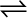7.5 Find out the value of Kc for each of the following equilibria from the value of Kp:

(i) 2NOCl (g)2NO (g) + Cl2 (g); Kp= 1.8 × 10–2 at 500 K

(ii) CaCO3 (s)CaO(s) + CO2(g); Kp= 167 at 1073 K

The relation between  is given as: